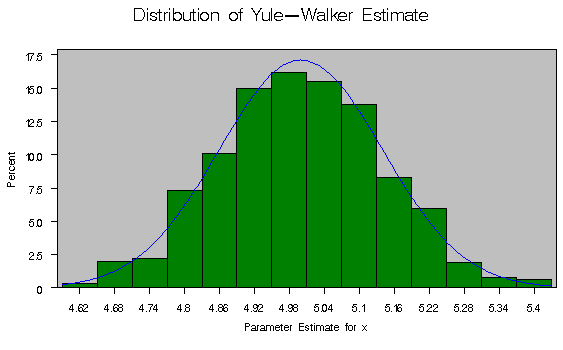SAS/ETS Examples

Efficiency Test for Estimators by Simulation

Contents | SAS Program

Overview

Econometricians are often interested in the finite sample efficiency of different estimators when the underlying assumptions of least squares break down. This specific example compares the efficiency of ordinary least squares (OLS) with that of the Yule-Walker method (often called Prais-Winsten) when disturbance terms are assumed to be first-order autocorrelated.

When first-order autocorrelation exists in OLS residuals, the OLS estimator is unbiased but not efficient. The sampling variances are underestimated, causing inferences from t- and F-tests to be invalid. One way to compensate for the autocorrelated residuals is the Yule-Walker method.The Yule-Walker method estimates the autoregressive form of the error term and then estimates the coefficients via generalized least squares (GLS). One particular advantage of the Yule-Walker method is that it retains the information in the first observation. This is crucial in small-sample estimation. The gain in efficiency can be investigated by performing a small Monte Carlo experiment.

The data are generated by the following AR(1) model:whereand ut are disturbances terms. Based on this model, this example compares the finite sample efficiency of OLS and the Yule-Walker method by drawing 1000 samples with 20 observations each.

Analysis

In order to compare the finite sample efficiency of OLS and the Yule-Walker method by simulation, this example generates 1000 samples of 20 observations each for the following model:This generation of samples is accomplished by using the DATA step to turn the model into simulated data. For this example, the value ofis set equal to 0.9. You can choose to repeat the experiment several times with other values ofto investigate the relative efficiency of the estimators in different error persistence environments.

data sample;
do samp = 1 to 1000;

rho = 0.9;

/* first error term */
eps = rho * rannor( 47392 ) + rannor( 82745 );

do x = 1 to 20;
y = 2 + 5 * x + eps;
eps=eps;
eps = rho * eps  + rannor( 32815 );
output;
end;
end;

OLS

Each sample is then estimated by OLS using the AUTOREG procedure to specify a linear regression of Y on X and a CONSTANT. The OUTEST= option creates the SAS data set EST0 that contains the parameter estimates from each of the samples. The NOPRINT option suppresses printed output. Estimates are computed for each sample by using the BY statement to specify BY-group processing.
proc autoreg data=sample outest=est0 noprint;
by samp;
model y = x ;
run;

The distribution of the parameter estimates can be calculated with the UNIVARIATE procedure. The following commands rename the two variables from the SAS data set EST0 and calculate their empirical distributions.

data estbar0;
set est0;
rename x=xbar0;
rename intercep=inter0;
run;

proc univariate data=estbar0;
var xbar0 ;
histogram xbar0 / normal(color=blue) cframe=ligr
cfill=green;
title 'Distribution of OLS Estimate';
run;

Below are the summary statistics for the coefficient of X. The mean is very close to 5, the true value, and the variance is 0.022508.

 Distribution of OLS Estimate

 The UNIVARIATE Procedure Variable: xbar0 (Parameter Estimate for x)

 Moments N 1000 Sum Weights 1000 Mean 5.00099049 Sum Observations 5000.99049 Std Deviation 0.15002553 Variance 0.02250766 Skewness 0.02338237 Kurtosis -0.2000697 Uncorrected SS 25032.391 Corrected SS 22.4851517 Coeff Variation 2.9999163 Std Error Mean 0.00474422

 Basic Statistical Measures Location Variability Mean 5.000990 Std Deviation 0.15003 Median 5.000203 Variance 0.02251 Mode . Range 0.86598 Interquartile Range 0.20464

 Tests for Location: Mu0=0 Test Statistic p Value Student's t t 1054.122 Pr > |t| <.0001 Sign M 500 Pr >= |M| <.0001 Signed Rank S 250250 Pr >= |S| <.0001

 Quantiles (Definition 5) Quantile Estimate 100% Max 5.45220 99% 5.36620 95% 5.24473 90% 5.19162 75% Q3 5.10437 50% Median 5.00020 25% Q1 4.89972 10% 4.81080 5% 4.75461 1% 4.65464 0% Min 4.58623Yule-Walker

The Yule-Walker estimator is calculated similarly. It is chosen as the method of estimation by specifying the NLAG= option in the model statement in the AUTOREG procedure. Again, the distribution of the parameter estimates is calculated by using the UNIVARIATE procedure.

proc autoreg data=sample outest=est1 noprint;
by samp;
model y = x / nlag=1;
run;

data estbar1;
set est1;
rename x=xbar1;
rename intercep=inter1;
run;

proc univariate data=estbar1;
var xbar1 ;
histogram xbar1 / normal(color=blue) cframe=ligr
cfill=green;
title 'Distribution of Yule-Walker Estimate';
run;

Like the OLS estimator, the mean of the Yule-Walker estimator is very close to 5. Notice, however, that the variance of the Yule-Walker estimator is 0.019559, which is less than that of the OLS estimator. Also, an examination of the quantiles reveals less dispersion in the Yule-Walker distribution as a whole.

 Distribution of Yule-Walker Estimate

 The UNIVARIATE Procedure Variable: xbar1 (Parameter Estimate for x)

 Moments N 1000 Sum Weights 1000 Mean 4.99968093 Sum Observations 4999.68093 Std Deviation 0.13985238 Variance 0.01955869 Skewness 0.02177098 Kurtosis -0.1709983 Uncorrected SS 25016.3485 Corrected SS 19.5391284 Coeff Variation 2.79722602 Std Error Mean 0.00442252

 Basic Statistical Measures Location Variability Mean 4.999681 Std Deviation 0.13985 Median 4.995825 Variance 0.01956 Mode . Range 0.83045 Interquartile Range 0.19028

 Tests for Location: Mu0=0 Test Statistic p Value Student's t t 1130.505 Pr > |t| <.0001 Sign M 500 Pr >= |M| <.0001 Signed Rank S 250250 Pr >= |S| <.0001

 Quantiles (Definition 5) Quantile Estimate 100% Max 5.42195 99% 5.34807 95% 5.21860 90% 5.17968 75% Q3 5.09476 50% Median 4.99582 25% Q1 4.90448 10% 4.81394 5% 4.77470 1% 4.67258 0% Min 4.59151Output

The output shows that, for this experiment, the Yule-Walker estimator is slightly more efficient than the OLS estimator. The results of the standard deviations of the distribution of the parameter estimates are s2yw = 0.019559 and s2ols = 0.022508 (where s2 denotes the variance). The fact that s2yw < s2ols implies greater efficiency for the Yule-Walker method.

An F-test can be computed to see if the variances are significantly different from each other. The F statistic is the ratio of the variances for the two estimators adjusted for degrees of freedom, which is 999 for both the numerator and the denominator. The variance of the OLS estimator, s2ols, is 0.022508, while the variance of the Yule-Walker estimator, s2yw, is 0.019559. Thus, the appropriate F statistic is given by

s2ols / s2yw = 0.022508 / 0.019559 = 1.1507746.

The p-value of this statistic for a one-sided test can be computed with the following DATA step

data _null_;
p = 1-probf( 1.1507746 , 999, 999 );
put p=;
run;

which returns a value of

1 - Pr F(1.1507746, 999, 999) = 0.013.

Thus, you would reject the null hypothesis that the efficiency of the two estimators is the same for most conventional levels of significance.

References

Greene, W.H. (1993), Econometric Analysis, Second Edition, New York: Macmillan Publishing Company.

Harvey, A.C. and McAvinchey, I.D. (1979), On the Relative Efficiency of Various Estimators of Regression Models with Moving Average Disturbances," Proceedings of the Econometric Society European Meetings, ed. E. Charatsis, Athens, 1979.

Johnston, J. (1972), Econometric Methods, Second Edition, New York: McGraw-Hill.

SAS Institute Inc. (1993), SAS/ETS User's Guide, Version 6, Second Edition, Cary, NC: SAS Institute Inc.